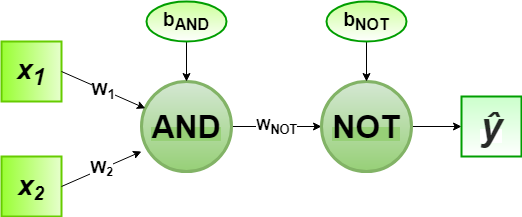# Implementation of Perceptron Algorithm for NAND Logic Gate with 2-bit Binary Input

In the field of Machine Learning, the Perceptron is a Supervised Learning Algorithm for binary classifiers. The Perceptron Model implements the following function:For a particular choice of the weight vectorand bias parameter, the model predicts outputfor the corresponding input vector.

NAND logical function truth table for 2-bit binary variables, i.e, the input vectorand the corresponding output0 0 1
0 1 1
1 0 1
1 1 0

We can observe that,Now for the corresponding weight vectorof the input vectorto the AND node, the associated Perceptron Function can be defined as:Later on, the output of AND nodeis the input to the NOT node with weight. Then the corresponding outputis the final output of the NAND logic function and the associated Perceptron Function can be defined as:For the implementation, considered weight parameters areand the bias parameters are.

Python Implementation:

 # importing Python library  import numpy as np     # define Unit Step Function  def unitStep(v):      if v >= 0:          return 1     else:          return 0    # design Perceptron Model  def perceptronModel(x, w, b):      v = np.dot(w, x) + b      y = unitStep(v)      return y     # NOT Logic Function  # wNOT = -1, bNOT = 0.5  def NOT_logicFunction(x):      wNOT = -1     bNOT = 0.5     return perceptronModel(x, wNOT, bNOT)     # AND Logic Function  # w1 = 1, w2 = 1, bAND = -1.5  def AND_logicFunction(x):      w = np.array([1, 1])      bAND = -1.5     return perceptronModel(x, w, bAND)     # NAND Logic Function  # with AND and NOT    # function calls in sequence  def NAND_logicFunction(x):      output_AND = AND_logicFunction(x)      output_NOT = NOT_logicFunction(output_AND)      return output_NOT     # testing the Perceptron Model  test1 = np.array([0, 1])  test2 = np.array([1, 1])  test3 = np.array([0, 0])  test4 = np.array([1, 0])     print("NAND({}, {}) = {}".format(0, 1, NAND_logicFunction(test1)))  print("NAND({}, {}) = {}".format(1, 1, NAND_logicFunction(test2)))  print("NAND({}, {}) = {}".format(0, 0, NAND_logicFunction(test3)))  print("NAND({}, {}) = {}".format(1, 0, NAND_logicFunction(test4)))

Output:

NAND(0, 1) = 1
NAND(1, 1) = 0
NAND(0, 0) = 1
NAND(1, 0) = 1


Here, the model predicted output () for each of the test inputs are exactly matched with the NAND logic gate conventional output () according to the truth table for 2-bit binary input.
Hence, it is verified that the perceptron algorithm for NAND logic gate is correctly implemented.

Attention geek! Strengthen your foundations with the Python Programming Foundation Course and learn the basics.

To begin with, your interview preparations Enhance your Data Structures concepts with the Python DS Course.

My Personal Notes arrow_drop_upCheck out this Author's contributed articles.

If you like GeeksforGeeks and would like to contribute, you can also write an article using contribute.geeksforgeeks.org or mail your article to contribute@geeksforgeeks.org. See your article appearing on the GeeksforGeeks main page and help other Geeks.

Please Improve this article if you find anything incorrect by clicking on the "Improve Article" button below.

Improved By : Akanksha_Rai